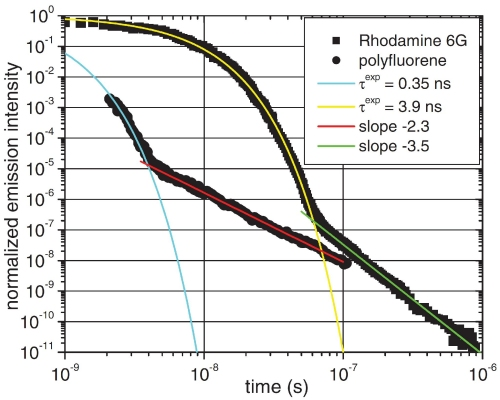# Exponential decay measurements using ICCD camera

C. Rothe et al., from the University of Durham determined with the 4-Picos intensified CCD camera the luminescence decay of dissolved organic materials over more than 20 lifetimes. They obtained the first experimental proof of the turnover into the non-exponential decay regime.The exponential decay of photon emitting dissolved organic molecules over 11 orders of magnitude intensity measured with the 4-Picos ICCD camera. The figure shows the first experimental proof of the turnover of a exponential decay into the non-exponential decay regime. Reprinted figure with permission from C. Rothe, S. I. Hintschich, and A. P. Monkman, Phys. Rev. Lett. 96, 163601 (2006). Copyright (2006) by the American Physical Society.

## First experimental proof of the turnover into the non-exponential decay

The exponential decay is a model which describes the decay of a certain number of subjects proportional to its total number. Many reactions and events in nature can be identified with exponential behavior. For example the radioactive decay can be described by the exponential decay law or the electric charge stored on a capacitor decays exponentially if the capacitor experiences a constant external load. The exponential decay is characterized either with the mean lifetime, which corresponds to the averaged time of all events, or the more intuitive half-life, which corresponds to the time when 50% of the total events happened.

### Limits of the exponential decay

However, in the mid of the 20th century, theoretical researcher found that true exponential function cannot fulfill certain mathematical boundary conditions. So they claimed that the exponential decay at some point must proceed more slowly. Certainly, this effect was predicted to be very small and would only be observable if only a fraction of the initial amount were present. Furthermore, experimental searches for the theoretical predicted deviation from the exponential decay have mainly focused on the decays of radioactive isotopes. It shows that the conditions for a reduction of the decay rate is very weak for samples with a large released energy compared to the width of the energy distribution. A theoretical approach suggested to use a range of atomic states including spontaneous photon emission or auto- and photo-ionizing states with small release energies. Hence, a range of organic molecules and polymers, dissolved in common solvents where chosen from C. Rothe et al. for their experiment. By using a ICCD camera, the exponential decay curves of these probes could be measured over 20-30 lifetimes.

### Measurements of exponential decay benefit from ICCD cameras

The sample is excited by a short laser pulse and the spectral dispersed emission is monitored by the high speed gated ICCD camera. The challenge of the exponential decay measurements is that the camera needs a ultra high shutter speed to provide good temporal resolution on the beginning of the decay. Furthermore, the detector needs to have extremely low noise contribution so the measurement is not affected by noise with the decreasing level of illumination.

The 4 Picos ICCD camera provides both, high shutter speeds with a gating time down to 200ps and extremely low noise with the possibility of single photon detection. Moreover, the within 10ps steps freely programmable delay time allows temporal scanning even of fast luminescence decays. With this performance the 4-Picos ICCD camera enables incredible 11 orders of magnitude intensity measurements as it can be seen in the figure above.